8129

Physics Waves And Oscillations Level: Misc Level

Earthquakes produce two kinds of seismic waves; The longitudinal primary waves(called p waves) and transverse secondary waves(called s waves).Both s waves and p waves travel through Earth,s crust and mantle, but at different speeds; the p waves are faster than s waves, but their exact speeds depend on depth and location.Assume the p waves speed to be vp=8580 m/s while the s waves travel at a slower speed of vs=5570 m/s. suppose a seismic station detects a wave and then ^t=93.5 s later detects an s wave.How far away is the earthquake center?Answer in units of km.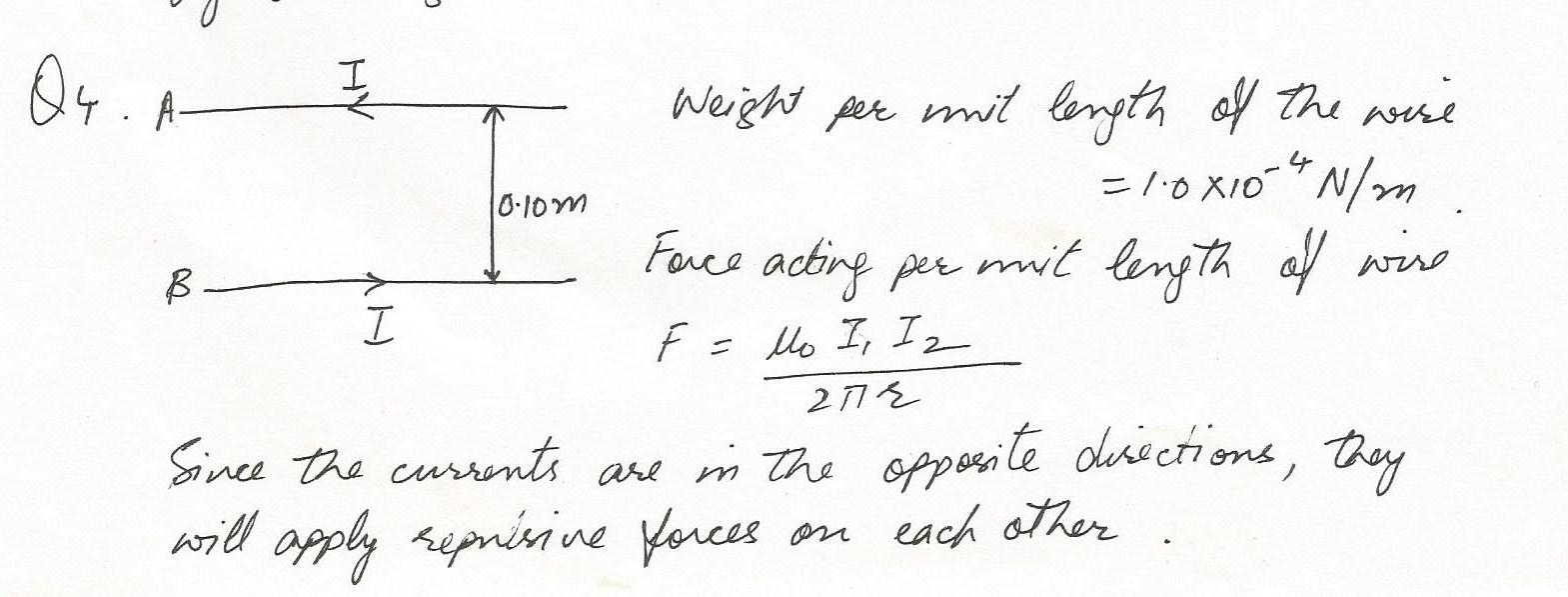8128

Physics Waves And Oscillations Level: Misc Level

What is the frequency of the wave?Answer in units of Hz.8127

Physics Waves And Oscillations Level: Misc Level

What is the period of the wave?Answer in units of s.7512

Physics Waves And Oscillations Level: Misc Level

A ship mapping the depth of the ocean emits a sound of 38 kHz. The sound travels to the ocean floor and returns 0.68 s later,A .)How deep is the water ar that location? B.)What is the waverngth of the wave in water?C.) What is the waverength of the retiected wave as it traveis into the air. where the speed of sound is 350 m/s?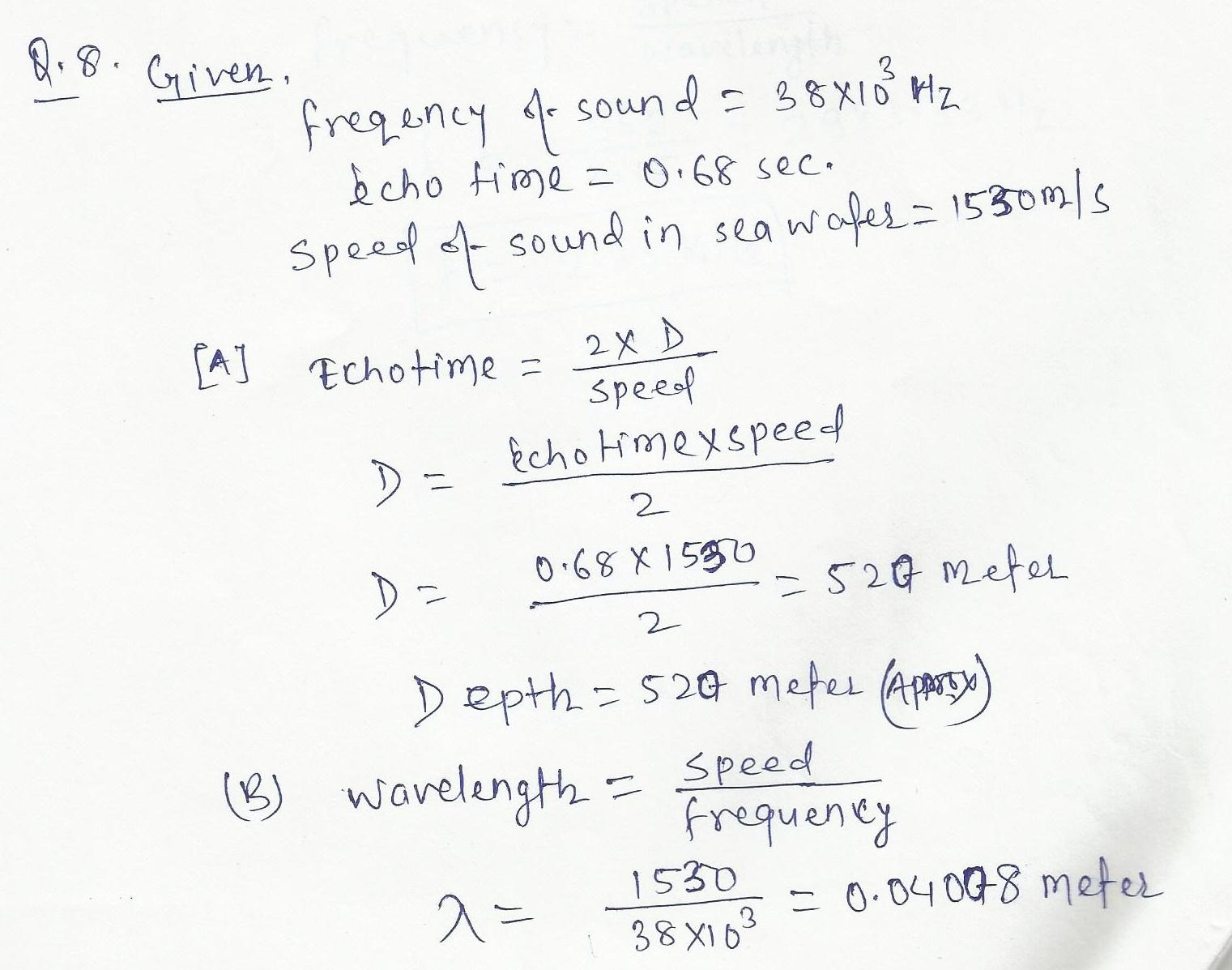7511

Physics Waves And Oscillations Level: Misc Level

A geoiogical survy ship mapping the floor of the ocean sends sound pulses down from the surface and measures the time taken for the echo to return.How deep is the ocean at a point wnere the echo time (down and back )is 7.07s7 The temperature or the seawater is 25 degree Ceisius.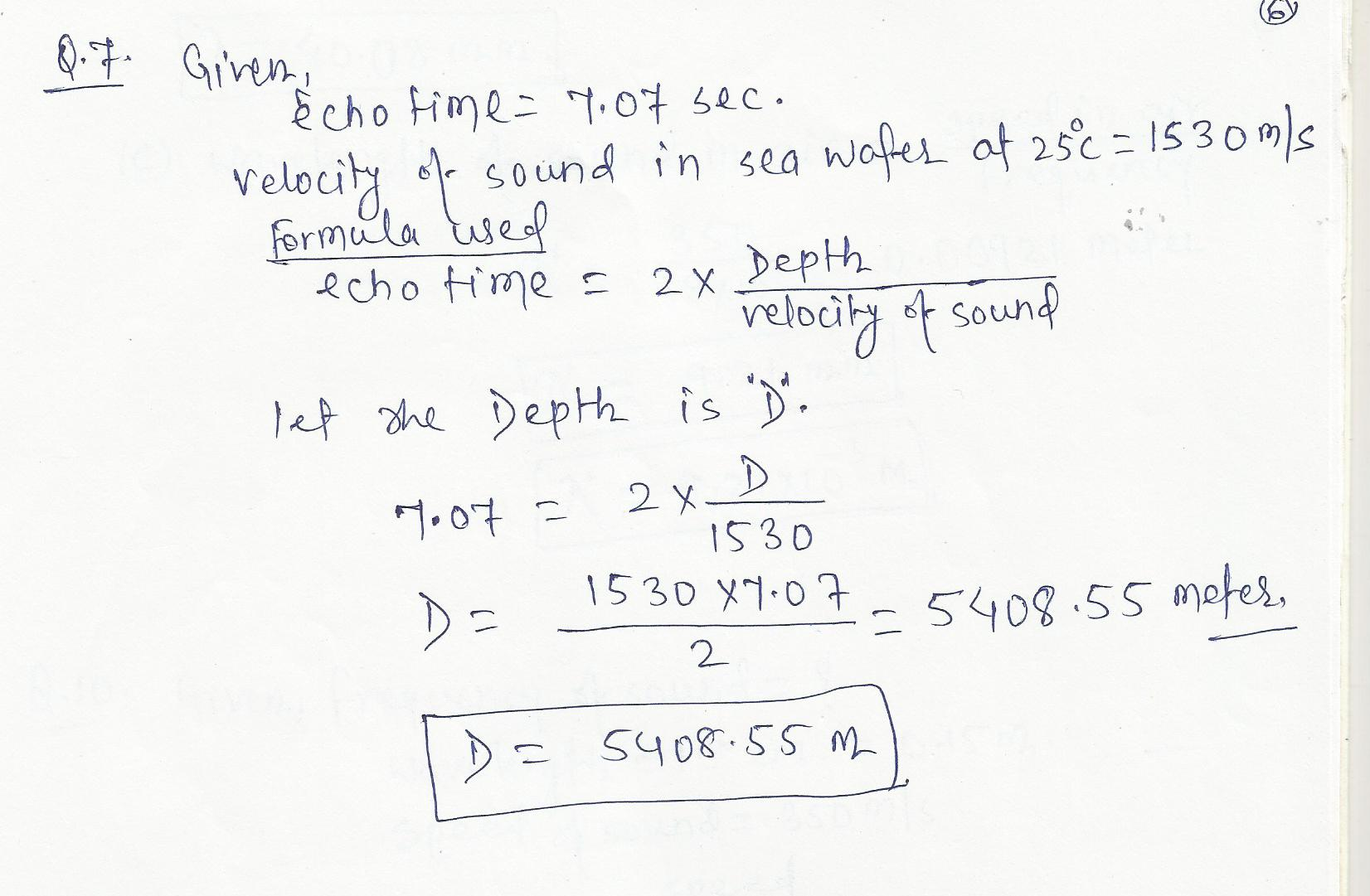7510

Physics Waves And Oscillations Level: Misc Level

A source of sound waves of frequency 1 kHz is traveling through the air at 0.50 times the speed of sound.A.) Find the frequency of the sound received by a stationary observer if the source moves toward her.B.)Repeat if the source moves away from her instead.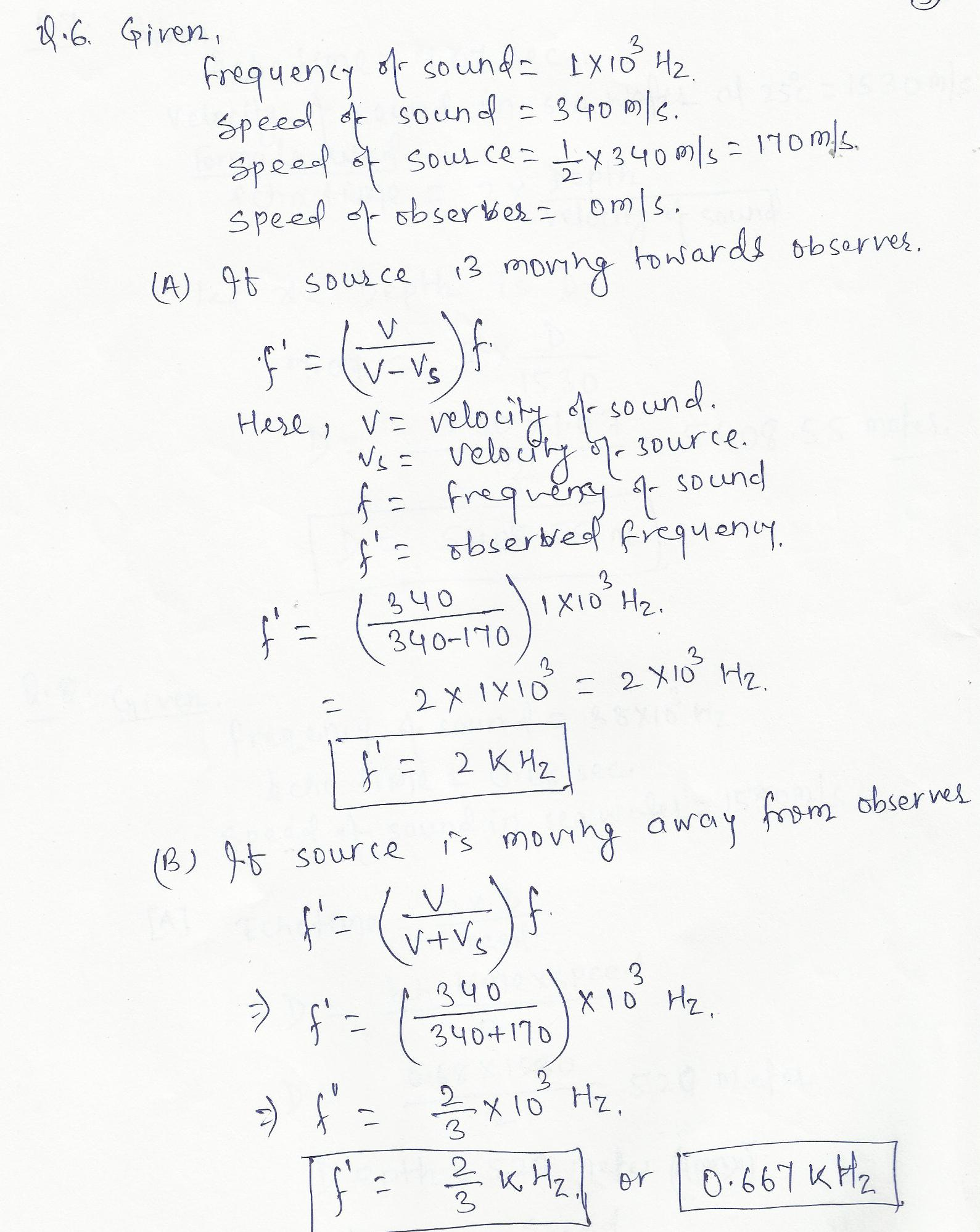7509

Physics Waves And Oscillations Level: Misc Level

At a factory, a noon whistle is sounding with a frequency of 500 Hz. As a car traveling at 85 km/h approaches the factory, the driver hears the whistle at frequency f initial. After driving past the factory, the driver hears frequency final. What is the change in frequency f final-f initial heard by the driver?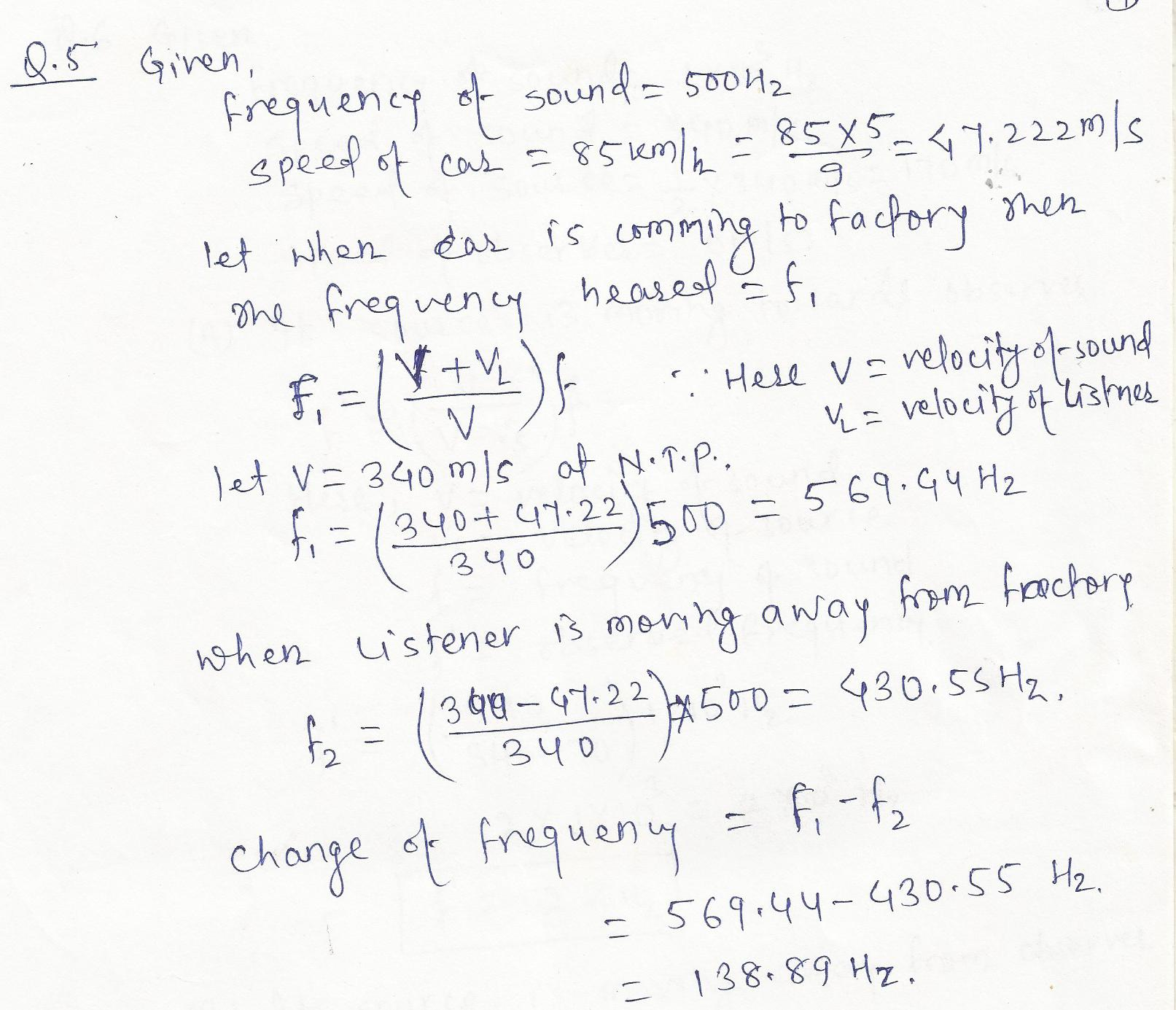7508

Physics Waves And Oscillations Level: Misc Level

A.) What is the pressure amplitude of a sound wave with an intensity level of 120 dB in air?B.) What force does this exert on an eardrum of area 0.550x10^-4 m^2?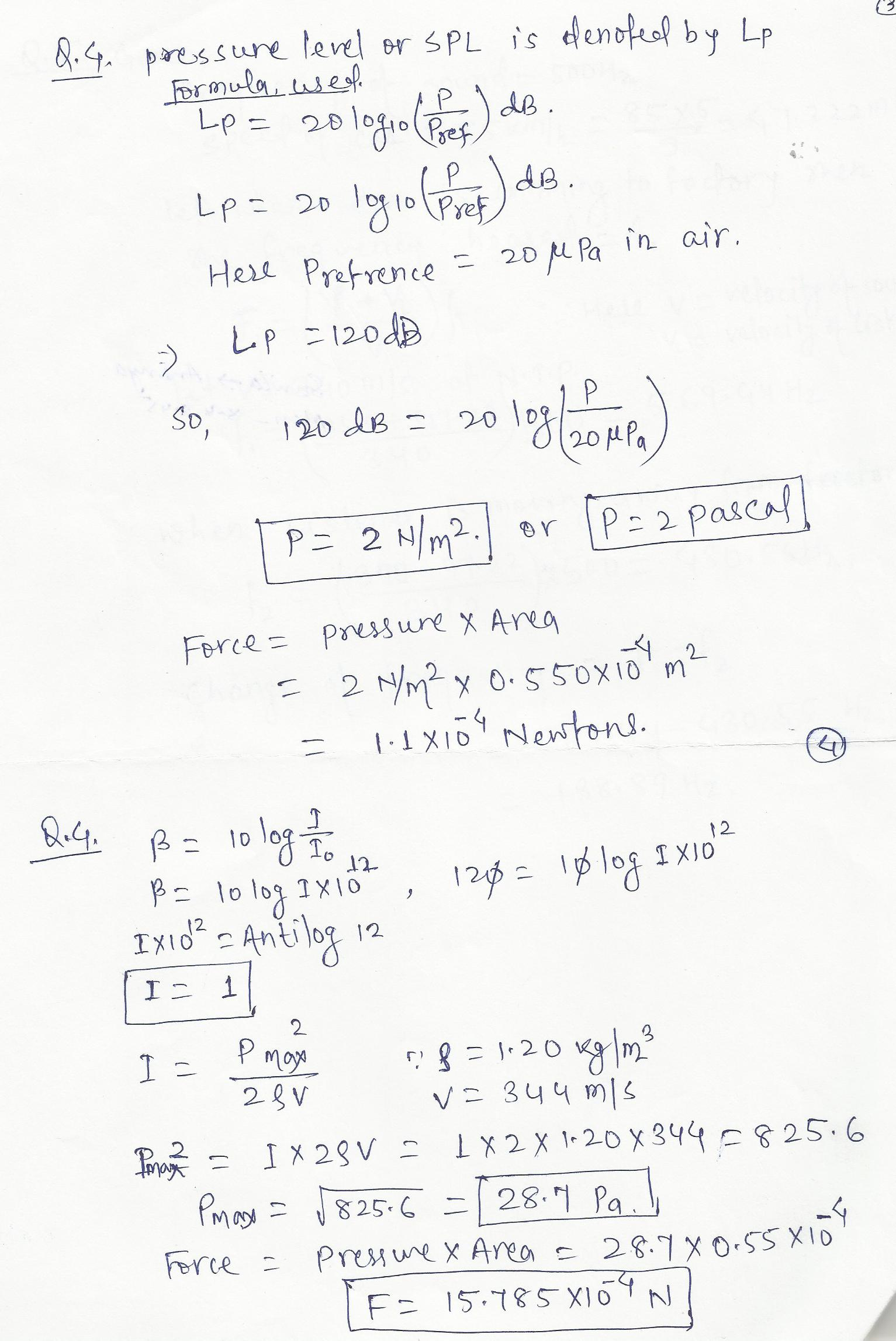7507

Physics Waves And Oscillations Level: Misc Level

A sound wave with an intensity level of 80 dB is incident on an eardrum of area 0.600 x 10^-4 m^2. How much energy is absorbed by the eardum in 3 minutes?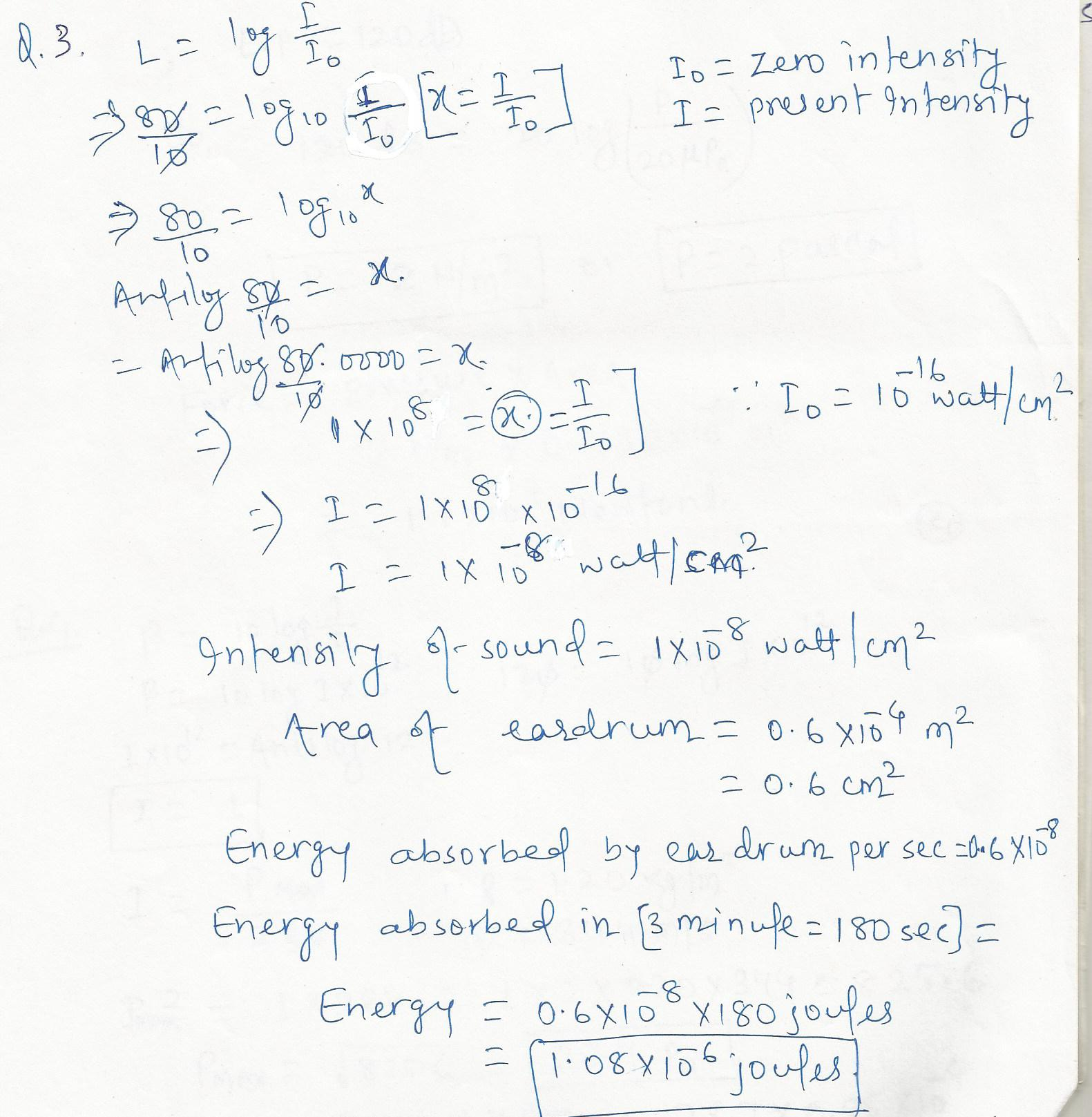7506

Physics Waves And Oscillations Level: Misc Level

A lightning flash is seen in sky and 8.2 s later the boom of the thunder is heard.The temperature of the air is 12 degree Celsius.A) What is the speed of sound at that temperature? (Light is an electromagnetic wave that travels at a speed of 3x10^8 m/s.) B.)How far away is the lightning strike?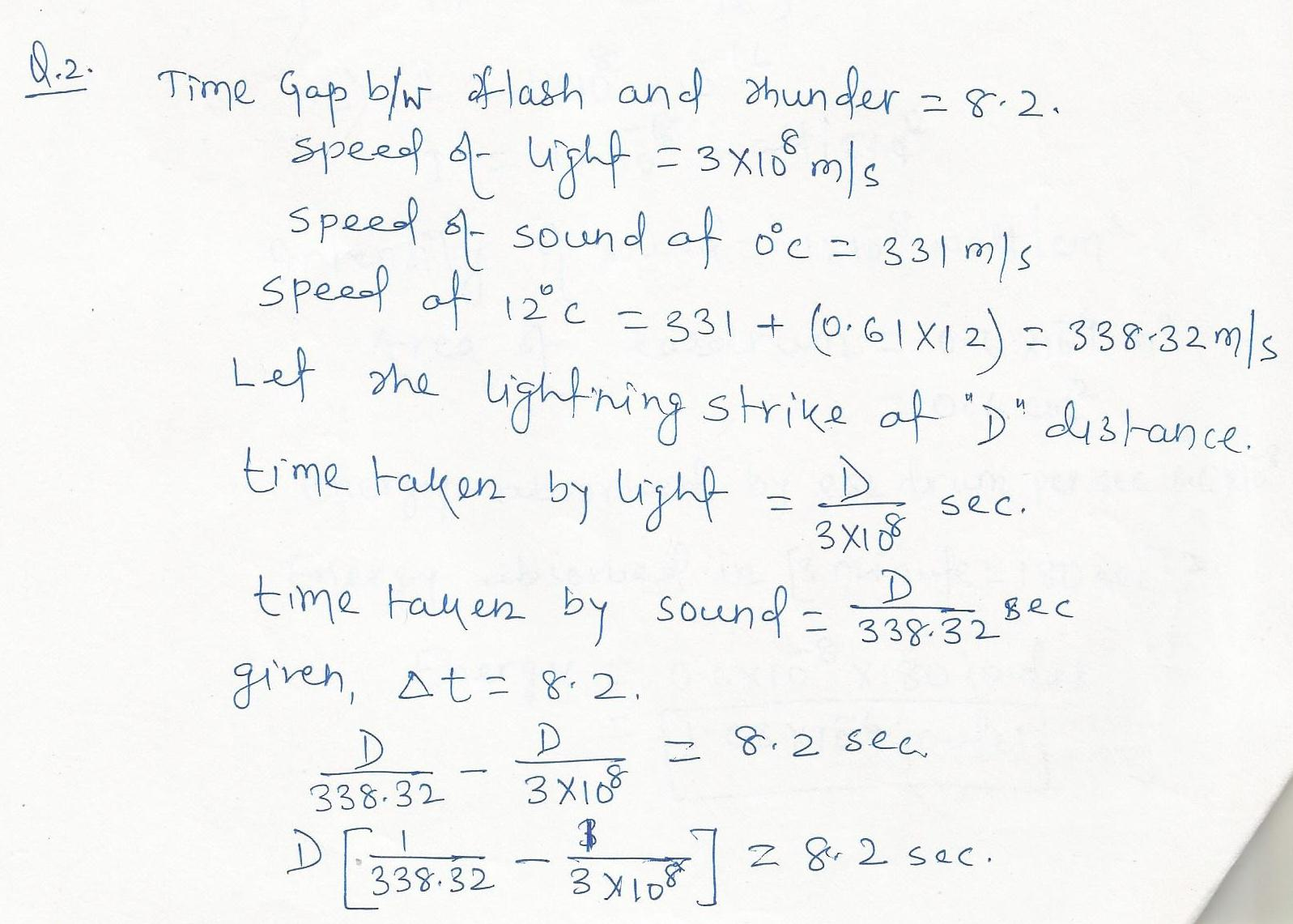7505

Physics Waves And Oscillations Level: Misc Level

Dolphins emit ultrasonic waves with a frequency as high as 2.5x 10^5 Hz.What is the wavelength of such a wave in seawater at 25 degree Celsius?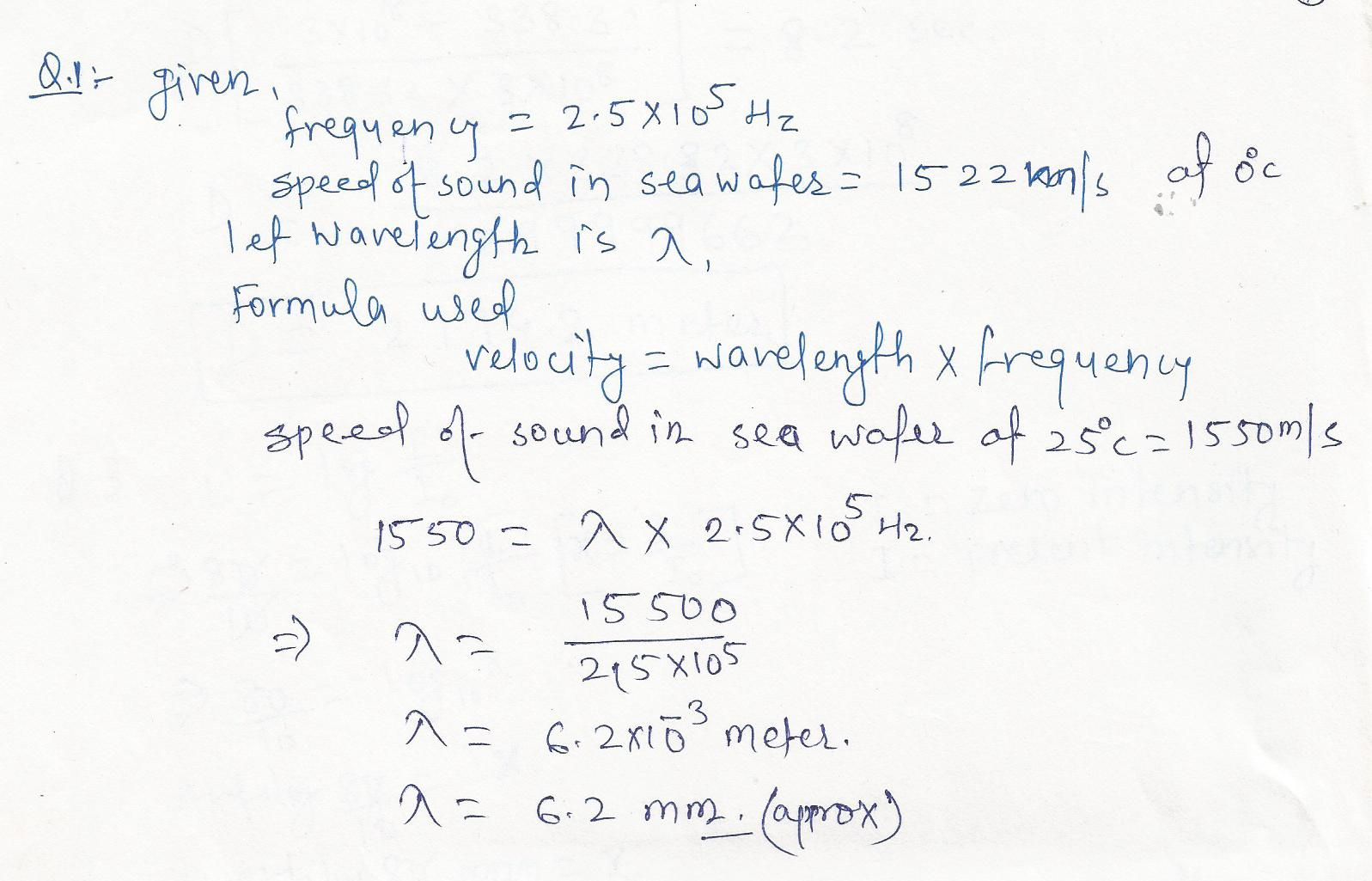7473

Physics Waves And Oscillations Level: Misc Level

Two identical tuning forks are struck, then one is placed on a moving stage, constant speed away from the observer, and the other is left stationary. What would the observer hear?Explain.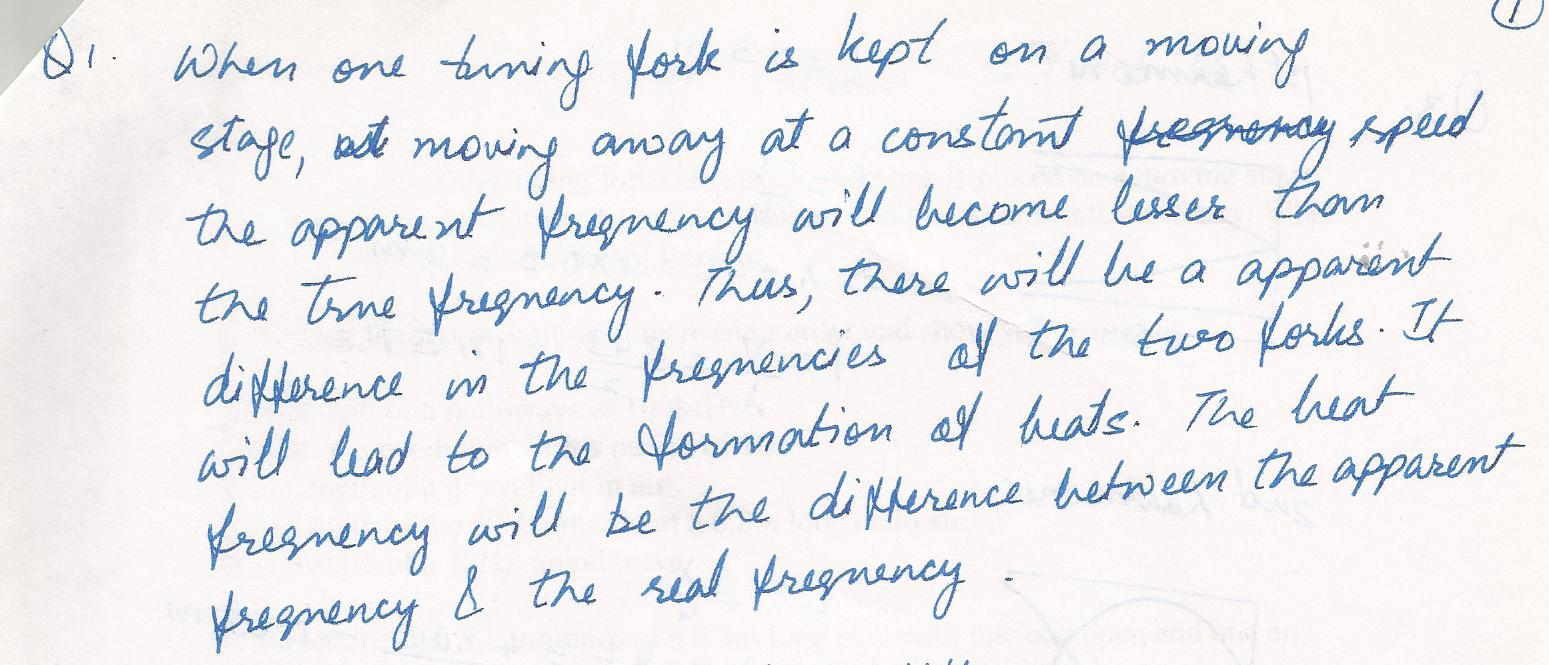7468

Physics Waves And Oscillations Level: Misc Level

tension is maintained in a string. The observed wave speed is 24 m/s when the suspended mass is 3.0 kg. a) what is the mass per unit length of the string? B) what is the wave speed when the suspended mass is 2.0 kg?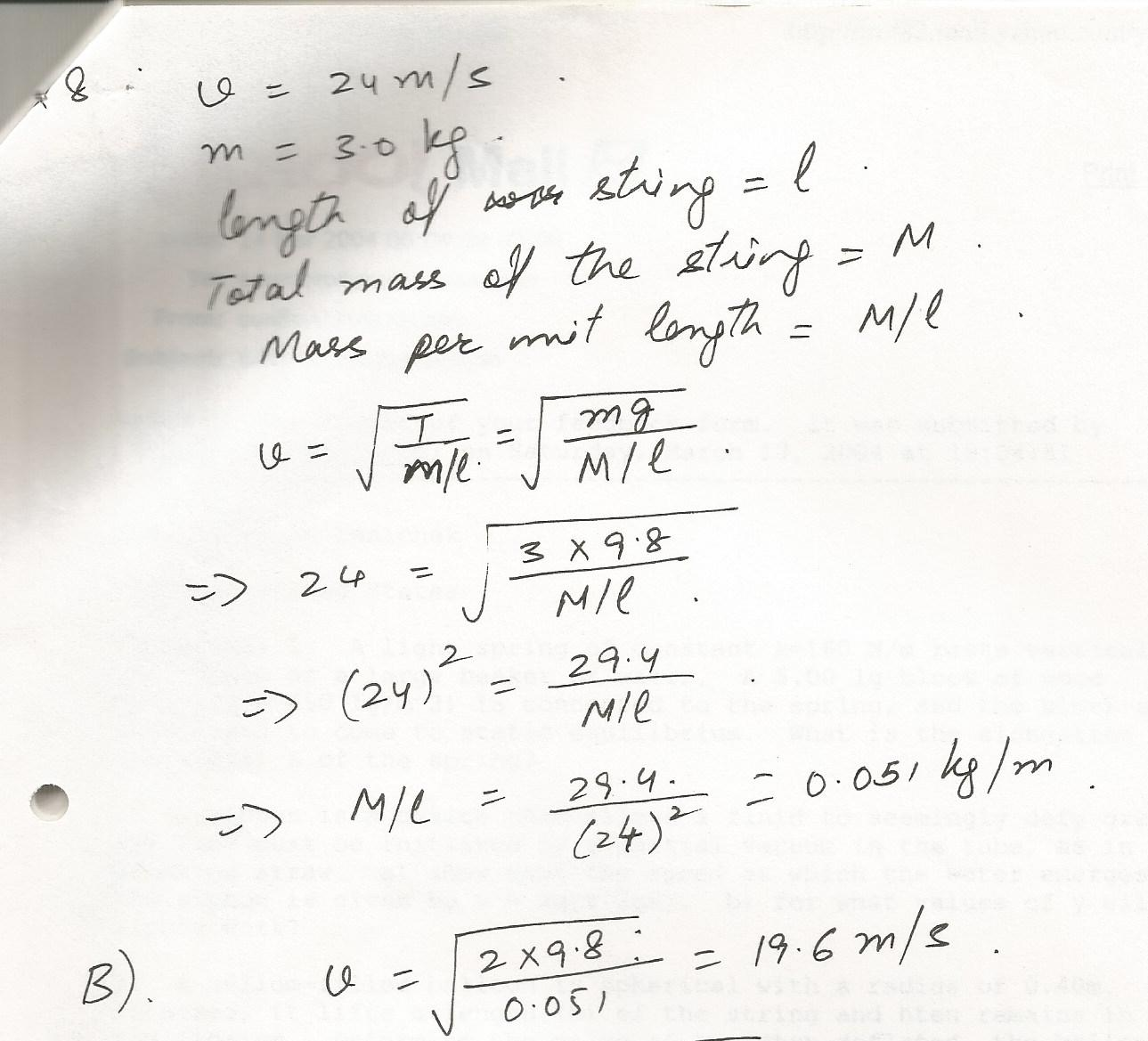7467

Physics Waves And Oscillations Level: Misc Level

A 1.5 kg block at rest on a tabletop is attached to a horizontal spring having constant 19.6 N/m. the spring is initially unstretched.cpnstant 20.0 N horizontal force is applied to the object causing the string to stretch. A) determine the speed of the object causing the moved 0.3m from equilibrium if the surface between the block and tabletop is frictionless. b) answer part  a) if the coefficient of kinetic friction between block and tabletop is 0.200.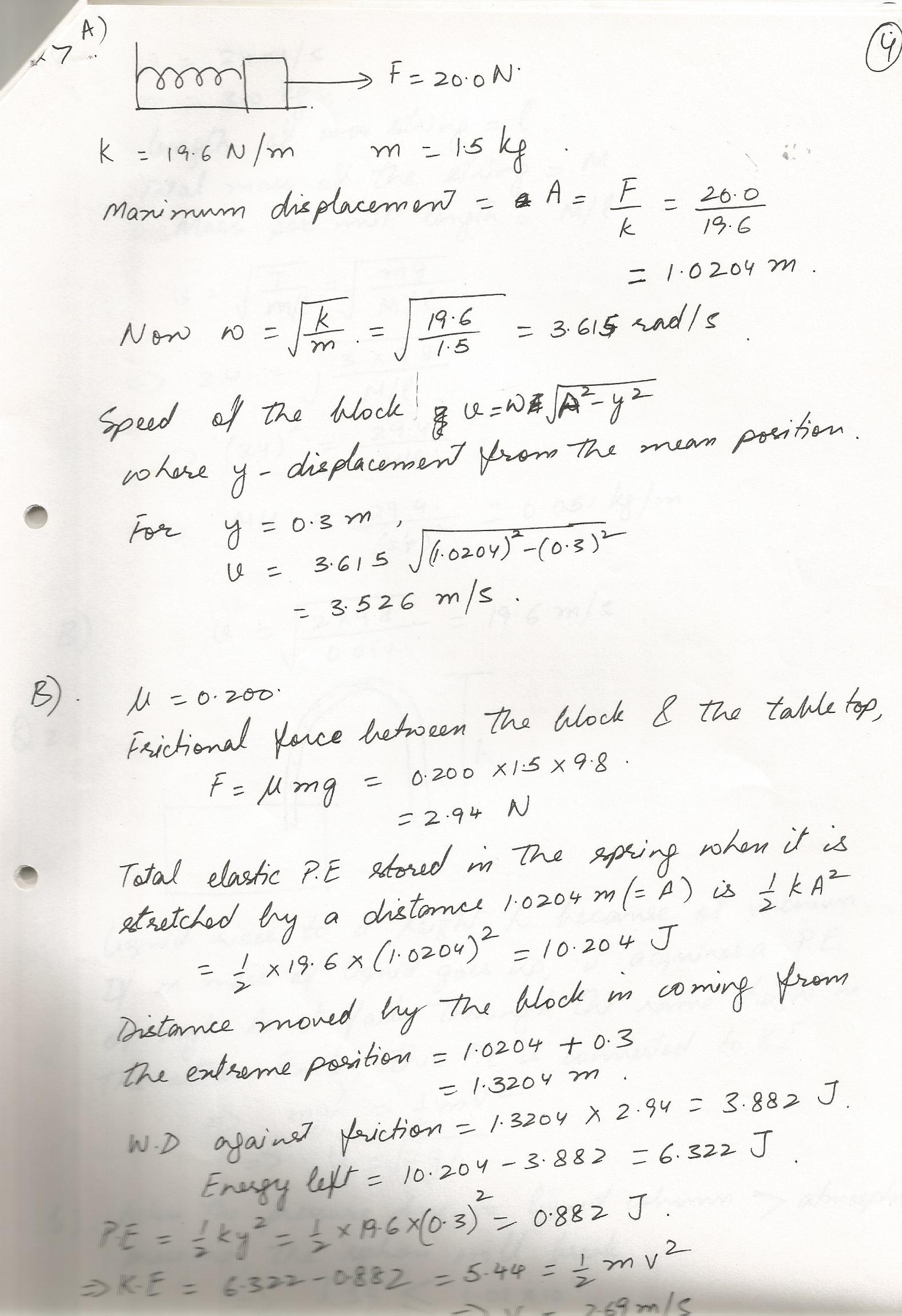7455

Physics Waves And Oscillations Level: Misc Level

Two identical tuning forks are struck, then one is placed on a moving stage, constant speed away from the observer, and the other is left stationary. What would the observer hear?Explain.Displaying 1-15 of 480 results.